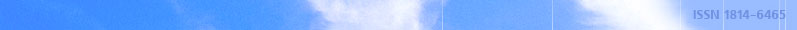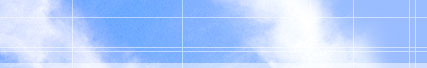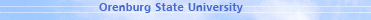december 04, 2021 RU/EN
 Headings of Vestnik Pedagogics Psychology Other

 Search Author Article

# March 2015, № 3 (178)

Rustanov A.R., Kharitonova S.V., Каzакоvа О.N. TWO CLASSES OF ALMOST C (λ)-MANIFOLDSAlmost contact metric manifolds have a rich differential geometric structures. The paper deals with almost contact metric manifolds, which are almost c (λ)-manifolds. D. Janssen and L. Vanhecke began investigated of almost c (λ)-manifolds. The curvature tensor is crucial for almost c (λ)-manifolds and curvature identities satisfied by this tensor are very important for understanding the differential geometric properties of almost c (λ)-manifolds. The results obtained in this paper identities expressing additional symmetry properties of the Riemannian curvature tensor of almost c (λ)-manifolds, allow to solve an actual problem of classifying almost c (λ)-manifolds, namely, to distinguish the class CR1 and CR2-class almost c (λ)-manifolds. We obtain the following identities curvature almost manifold. Key words: almost c (λ)-manifold, cosymplectic manifold, c (λ)-manifold, the Riemannian curvature tensor, Sasakian manifold, manifold Kenmotsu.

References:

1. Janssen, D. Almost contact structures and curvature tensors / D. Janssen, L. Vanhecke // Kodai Math. J. — № 4. — 1981. — P.1-27.

2. Olszak, Z. Normal locally conformal almost cosymplectic manifolds / Z. Olszak, R. Rosca // Publ. Math. Debrecen. — 39:3-4. — 1991. — P.315-323.

3. Kharitonova, S.V. Almost C(l) manifolds / S.V.Kharitonova //, Journal of Mathematical Sciences, 177(2011), 742-747.

4. Akbar, А.Some Results on Almost manifolds / Ali Akbar // International Journal of Mathematical Sciences Engineering and applications (IJMSEA). — 7:1. — 2013. — Р.255-260.

5. Akbar, А. On the Conharmonic and Concircular curvature tensors of almost manifolds / Ali Akbar, Avijit Sarkar // International Journal of Advanced Mathematical Sciences (IJMSEA). — 1:3. — 2013. — Р.134-138.

6. Kirichenko, V. F. Differential-Geometric Structures on Manifolds / V.F.Kirichenko. — Moscow.: MSPU. — 2003. — 495p.

7. Kirichenko, V. F. Differential geometry of quasi-Sasakian manifolds / V.F.Kirichenko, A.R. Rustanov // Matematicheskiy Sbornik — 2002. — Vol.8. — N193. — P.1173–1201.

8. Rustanov, A.R. Curvature identities manifolds of class С11 / A.R.Rustanov, N.N.Shchipkova // Vestnik of Orenburg State University. №6, 2011, p. 169-171.

9. Rustanov, A.R. Geometry curvature tensor concircular AC-manifolds of class С11 / A.R.Rustanov, N.N.Shchipkova // Vestnik of Orenburg State University. №9, 2014, P. 114-120.Editor-in-chief Sergey Aleksandrovich MIROSHNIKOV